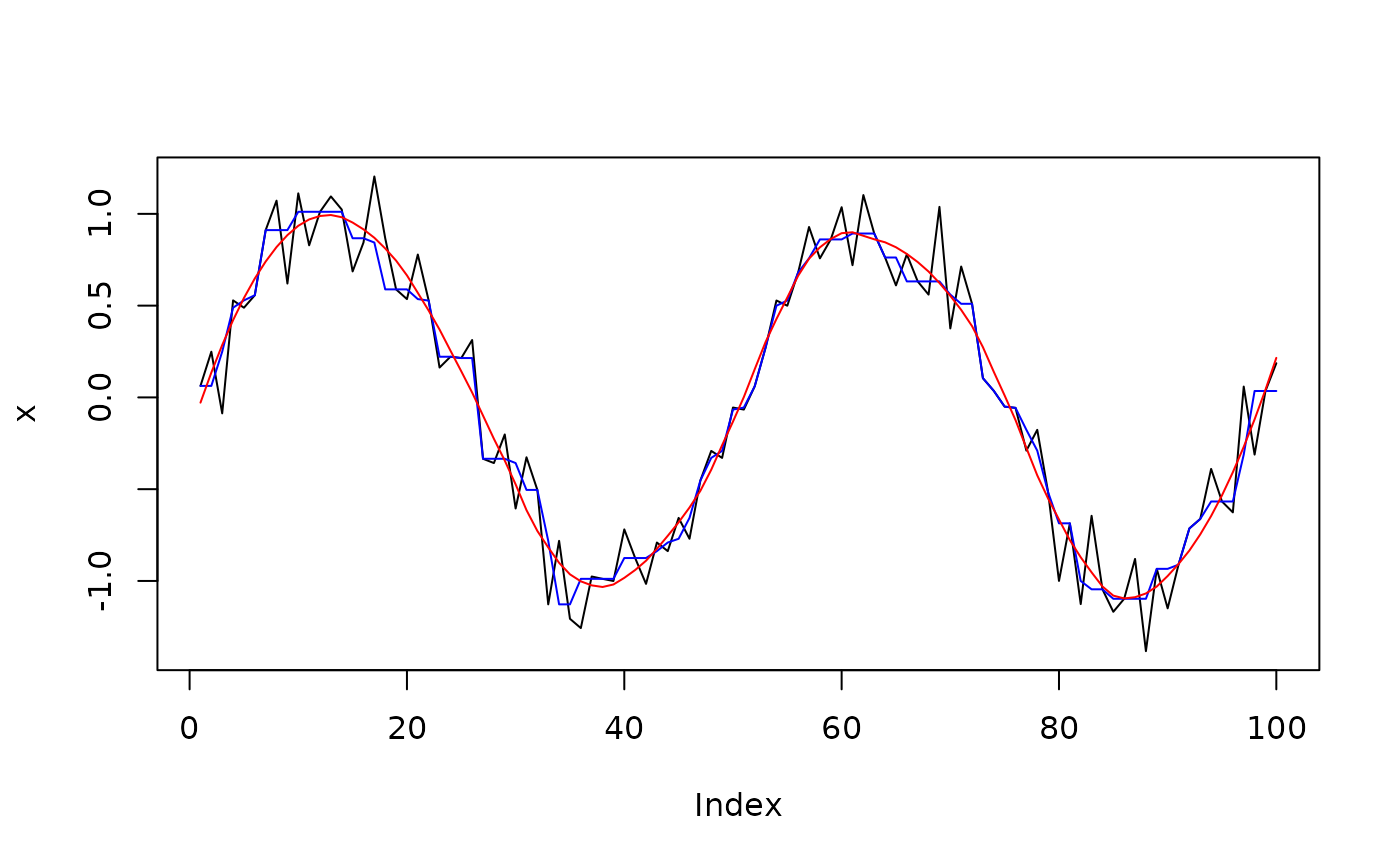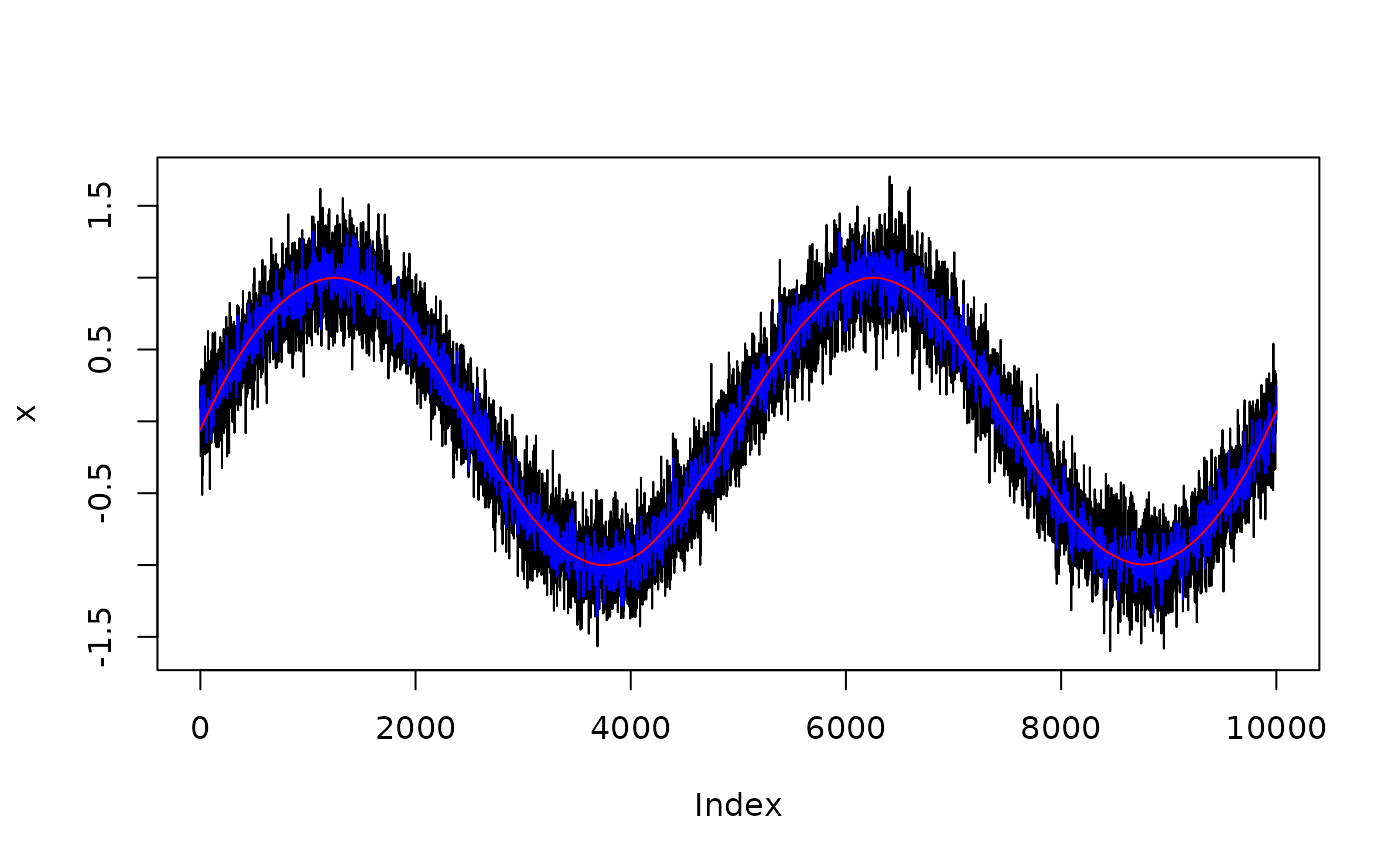Smoothing a vector or a time series. For data.frames, the function will smooth all numeric variables stratified by factor levels (i.e., will smooth within each factor level combination).

## Usage

smoothing(x, method = "loess", strength = 0.25, ...)

## Arguments

x

A numeric vector.

method

Can be "loess" (default) or "smooth". A loess smoothing can be slow.

strength

This argument only applies when method = "loess". Degree of smoothing passed to span (see loess()).

...

Arguments passed to or from other methods.

## Value

A smoothed vector or data frame.

## Examples

x <- sin(seq(0, 4 * pi, length.out = 100)) + rnorm(100, 0, 0.2)
plot(x, type = "l")
lines(smoothing(x, method = "smooth"), type = "l", col = "blue")
lines(smoothing(x, method = "loess"), type = "l", col = "red")x <- sin(seq(0, 4 * pi, length.out = 10000)) + rnorm(10000, 0, 0.2)
plot(x, type = "l")
lines(smoothing(x, method = "smooth"), type = "l", col = "blue")
lines(smoothing(x, method = "loess"), type = "l", col = "red")